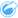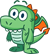# Predict Variable

Predict Variable uses a regression model to predict new values in a dataset. Predict Variable must be used in conjunction with Create Regression Model.

## Example

An environmental organization is trying to predict the future greenhouse gas emissions by country until 2050. An analyst from the organization created a regression model using population and gross domestic product (GDP) with Create Regression Model. The analyst can then use the model and projected population and GDP values to predict the future greenhouse gas emissions per country.

## Use the Predict Variable capability

Use the following steps to run the Predict Variable analysis capability:

1. Create a map, chart, or table using the dataset with which you want to predict a variable.
2. Click the Action button.
3. Do one of the following:
• If your card is a chart or table, click How is it related? in the Analytics pane.
• If your card is a map, click the Find answers tab and click How is it related?.
4. Click Predict Variable.
5. For Choose a layer, select the dataset with which you want to predict a variable.
6. For Choose the regression model layer, select the function dataset you want to use as a model. A function dataset must be created using Create Regression Model.
7. For Map variables, select the fields in your input layer that correspond to the explanatory variables in the regression model.
8. Click Run.

A dataset is created with the estimated values based on your regression model and input variables.

##### Tip:You can also open the Predict Variable pane by dragging a function dataset onto a map card.

## Usage notes

Predict Variable can be found using the Action buttonunder How is it related? on the Find answers tab. You can also open the Predict Variable pane by dragging a function dataset onto a map card.

You must choose a regression model in the form of a function dataset to use Predict Variable. A model can be created using Create Regression Model. The equation and statistics for the model are given on the dialog box.

The Map variables parameter is used to match explanatory variables from the regression model to fields in the input dataset.